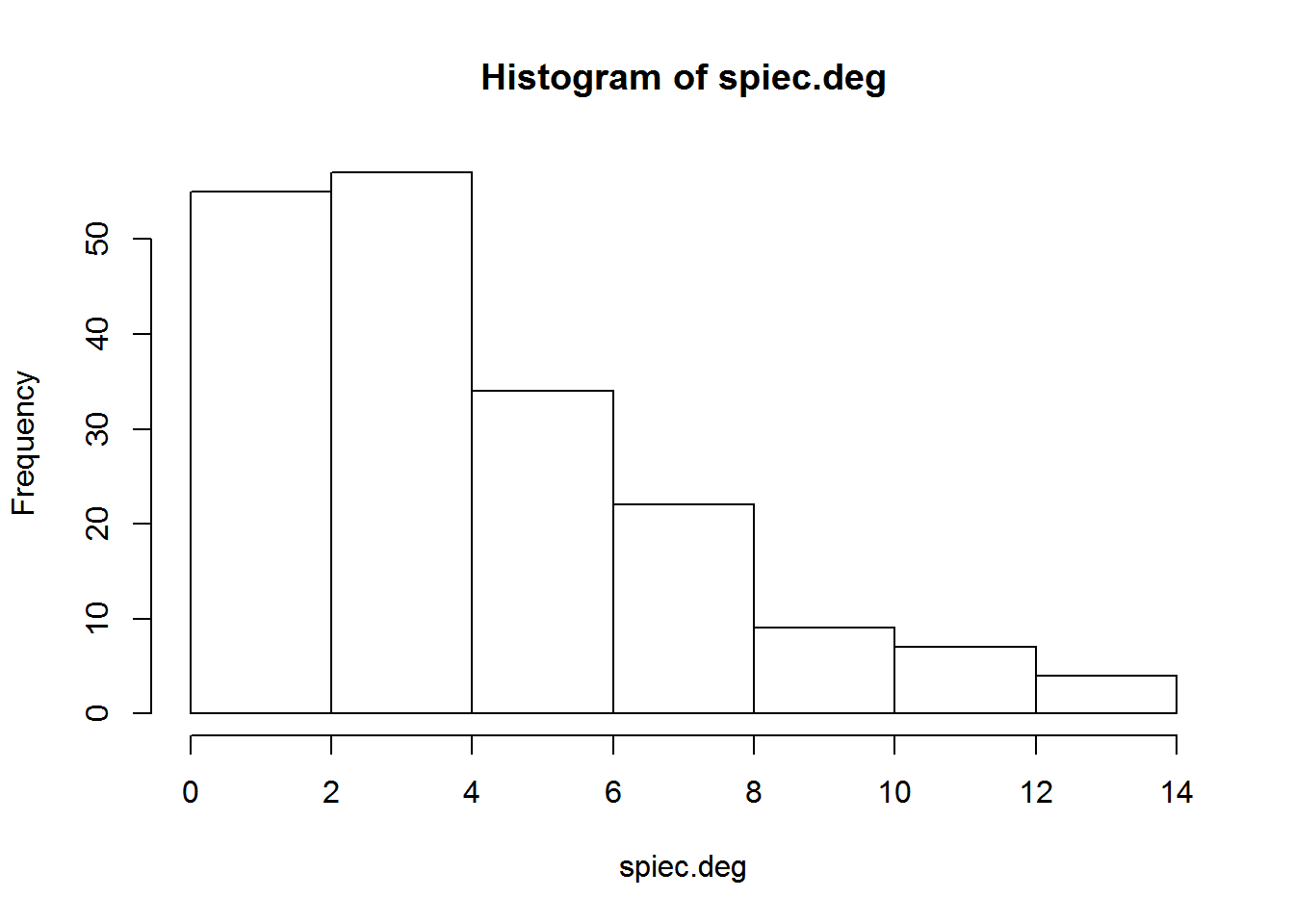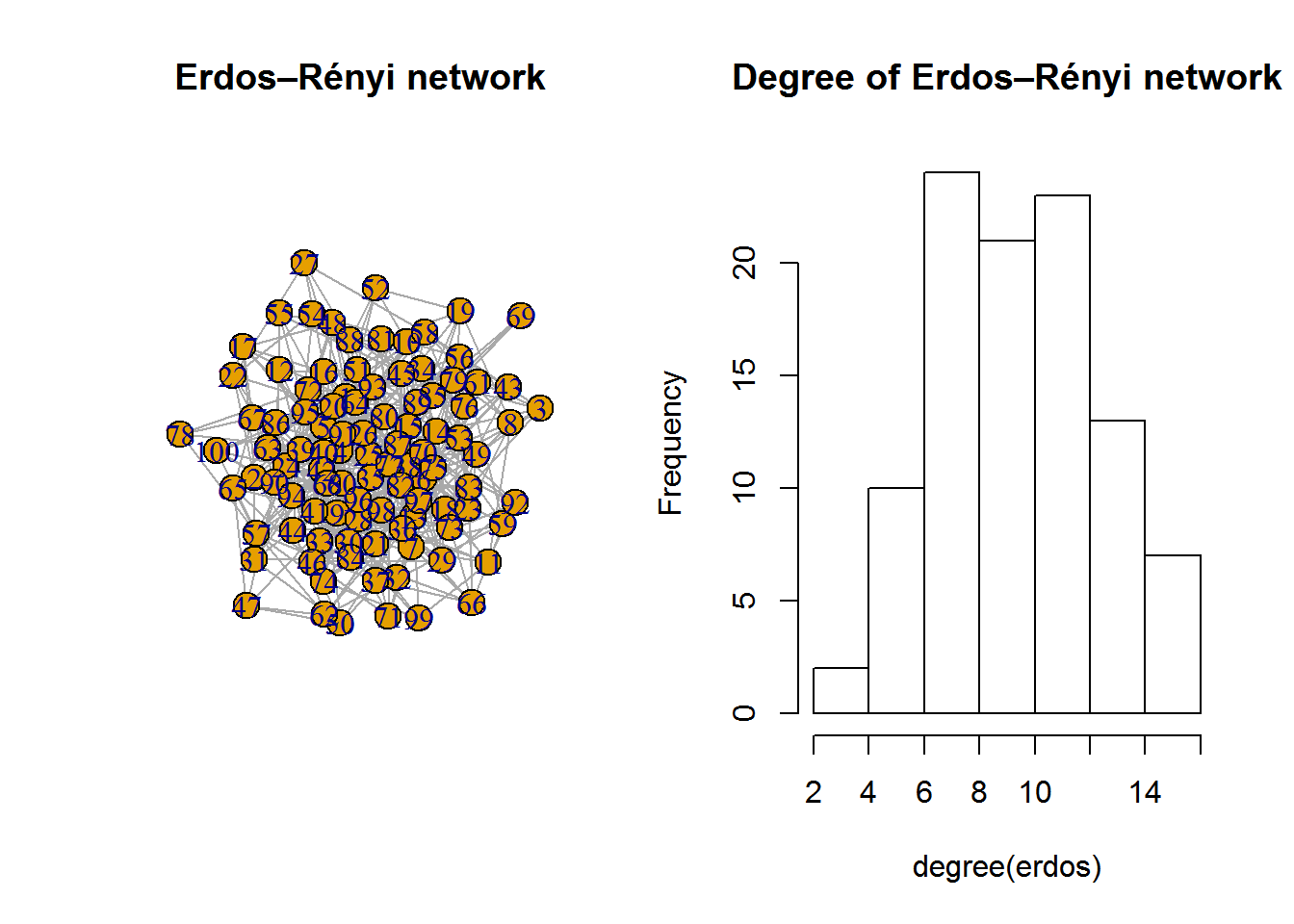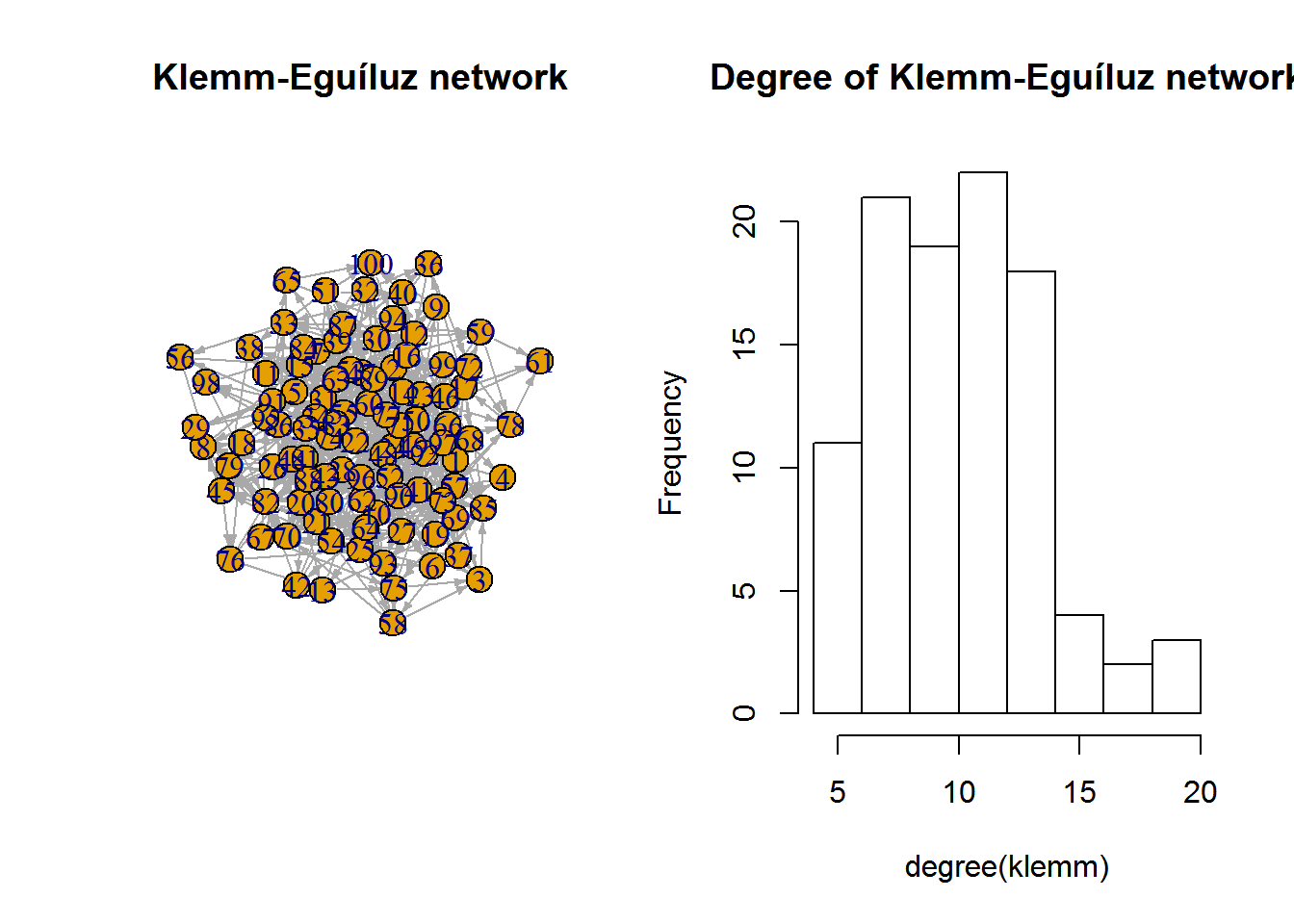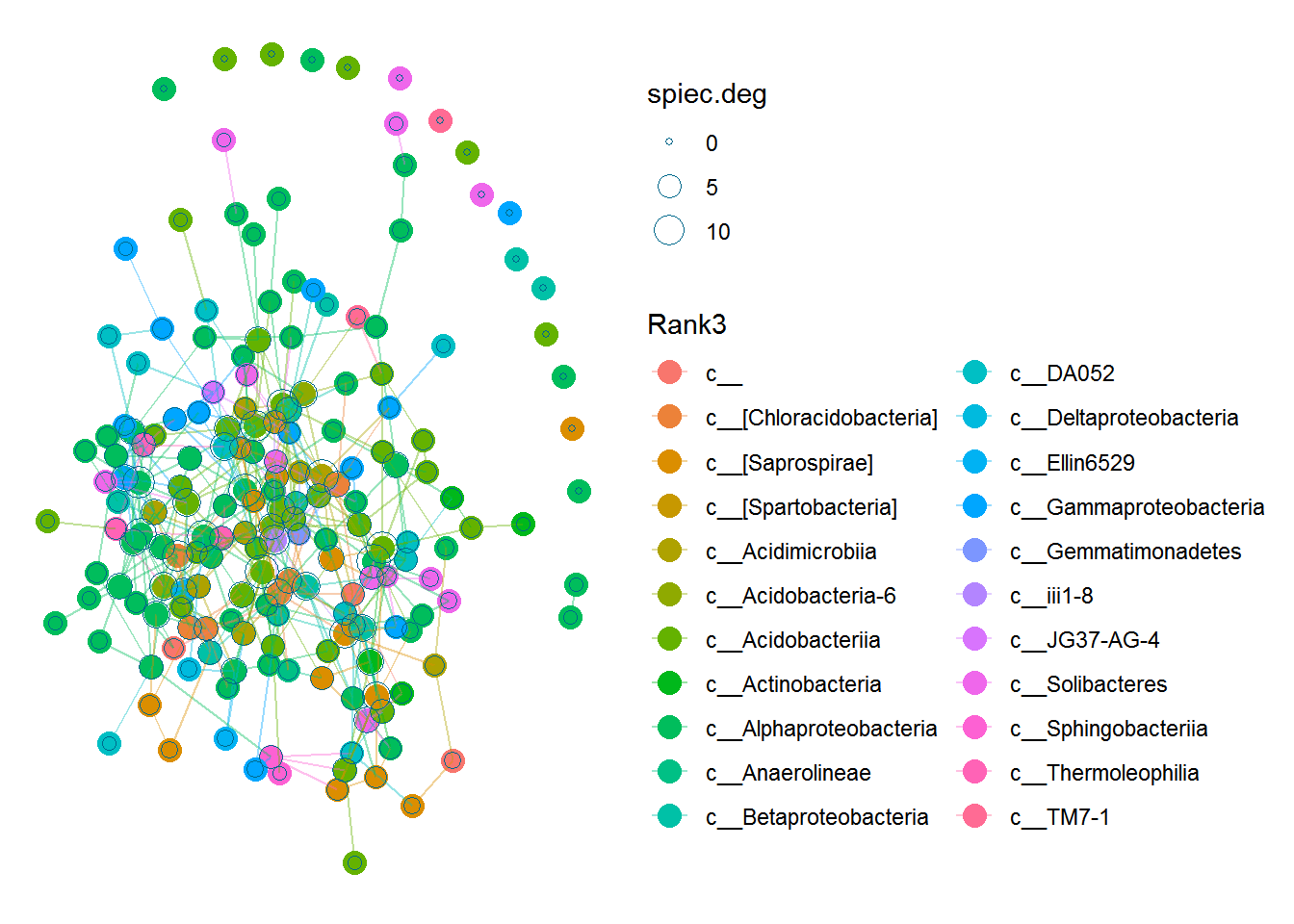# Network analysis - node importance

Previously, we looked at hub nodes; the nodes with the largest number of connections. However, there are many other ways to define node centrality or to look at properties of individual nodes. While there are apps available in Cytoscape (e.g. CytoNCA), we are going to analyse our SPIEC-EASI network with the igraph library.

## Step 1 - Data

We are going to work with the arctic soils network generated by SpiecEasi. If you have not downloaded the network yet, get the files here and load it into an igraph object. We are going to need the estimated edge weights for some analysis steps. Don’t set the ‘weight’ property of the graph to the edge weights, as this breaks some igraph functions.

``````library(SpiecEasi)
library(phyloseq)
library(ggplot2)
library(igraph)
library(seqtime)

### If you can't import the file:
### They are in the Workshop folder
### This file is already preprocessed!
phyloseqobj.f <- phyloseq(otu_table(otus, taxa_are_rows = TRUE), tax_table(tax))
# use your SPIEC-EASI graph from the previous exercise
# or load the igraph graphml file supplied in the github repo

## Step 2 - Network centralities

One of the simplest network centralities is degree centrality, where the degree is the number of connections a node has. We can use a hist to visualize node degree. If the degree distribution of a network follows a power law, that network is scale-free. We can fit a power law to the network with the fit_power_law function. Do you think this is a scale-free network based on those outcomes?

``````spiec.deg <- degree(spiec.graph)
hist(spiec.deg)````````````fit <- fit_power_law(spiec.deg)
fit``````
``````## \$continuous
##  FALSE
##
## \$alpha
##  4.441027
##
## \$xmin
##  7
##
## \$logLik
##  -81.70369
##
## \$KS.stat
##  0.07647556
##
## \$KS.p
##  0.9666786``````

Microbial networks do not always follow a power law. However, the extent to which they do may have important biological implications. Can you explain why a scale-free network would be less robust to failure than other networks? You can find the solutions here. We can generate networks with the igraph and seqtime libraries that have different degree distributions. The Erdős–Rényi model generates completely random networks, while the Klemm-Eguíluz algorithm generates networks that are scale-free. Try removing random edges from these models to see this affects connectivity.``````##  "Adjusting connectance to 0.1"
##  "Initial edge number 10000"
##  "Initial connectance 1"
##  "Number of edges removed 8910"
##  "Final connectance 0.1"
##  "Final connectance: 0.1"``````To better visualize our association network, we can plot the degree as node size in the network. Because the object returned by plot_network is a regular ggplot object, we can overlay circles that indicate the degree of each node. Species with the largest degree in a network are often called hub species.

``plot_network(spiec.graph, phyloseqobj.f, type='taxa', color="Rank3", label=NULL) + geom_point(aes(size=spiec.deg), colour='deepskyblue4', shape=1)``In addition to degree, betweenness centrality is often used. The betweenness centrality is determined by the number of shortest paths passing through a node, where the shortest paths are from all nodes to all other nodes. Try plotting the betweenness centrality yourself; can you explain why there are no nodes with high betweenness centrality but low degree? Try drawing a network that does have these nodes. For solutions, check here.

Like betweenness centrality, closeness centrality uses shortest paths. However, instead of counting the total number of shortest paths passing through a node, this centrality measure uses the shortest paths from the node in question to all other nodes. Therefore, it measures how distant a node is to other nodes. Closeness centrality only makes sense for graphs with a single connected component, as there are no shortest paths from one part of the network to a disconnected part. This is also visible in the histogram and the graph: igraph estimated very low closeness centrality for these disconnected nodes. Try generating a better visualization of the closeness centrality. Do you have an idea of the nodes in the central component that will have the lowest closeness centrality? Answers are here.

``spiec.close <- closeness(spiec.graph)``

A different type of centrality is eigenvector centrality. This measure takes the set of neighbours of a node and uses those to calculate the node’s eigenvector.

``````spiec.eigen <- eigen_centrality(spiec.graph)
hist(spiec.eigen\$vector)``````